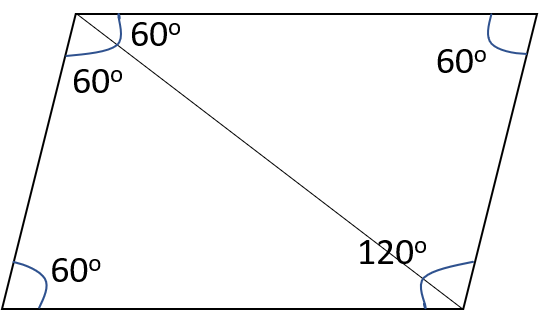# CAT Quantitative Aptitude Questions | Geometry Questions for CAT - Triangles

###### CAT Questions | CAT Geometry Questions | Quadrilateral

The question is from the topic quadrilaterals. In this problem perimeter and area of a rhombus are given and we have to find out the sum of the lengths of its diagonals. CAT Geometry questions are heavily tested in CAT exam. Make sure you master Geometry problems. It uses the idea of area of rhombus is equal to the sum of the triangles contained in it.

Question 17: Rhombus has a perimeter of 12 and one angle = 120°. Find its area.

1. 9 * (√3)/2
2. 3 * (√3)/2
3. 9 * √3
4. 18 * √3

## Best CAT Coaching in Chennai

#### CAT Coaching in Chennai - CAT 2022Limited Seats Available - Register Now!

##### Method of solving this CAT Question from Quadrilaterals: Knowing the properties of rhombus helps.Perimeter = 12.
Let the side of the rhombus be “a�?, then 4a = 12 => a = 3.
One angle = 120°.
Adjacent angles of a rhombus are supplementary. Therefore, the other angle = 60°.
Diagonals of a rhombus bisect each other, therefore, ∠DAC = 60° ∠DCA =60° ∠BAC =60°
Therefore, Triangle DAC and BAC are equilateral triangles.
Therefore, Area of Rhombus = 2 * Area of the Equilateral Triangle
= 2 * (√3/4) * a2 = (√3/2) * 9 = 9 * (√3/2).

The question is " Find the area of the rhombus"

##### Hence, the answer is 9 * (√3/2).

Choice A is the correct answer.

###### CAT Coaching in ChennaiCAT 2023

Classroom Batches Starting Now! @Gopalapuram

###### Best CAT Coaching in Chennai Introductory offer of 5000/-

Attend a Demo Class

## CAT Preparation Online | CAT Geometry questions Videos On YouTube

#### Other useful sources for Geometry Question | Geometry Triangles Circles Quadrilaterals Sample Questions

##### Where is 2IIM located?

2IIM Online CAT Coaching
A Fermat Education Initiative,
58/16, Indira Gandhi Street,
Kaveri Rangan Nagar, Saligramam, Chennai 600 093

##### How to reach 2IIM?

Phone: (91) 44 4505 8484
Mobile: (91) 99626 48484
WhatsApp: WhatsApp Now
Email: prep@2iim.com